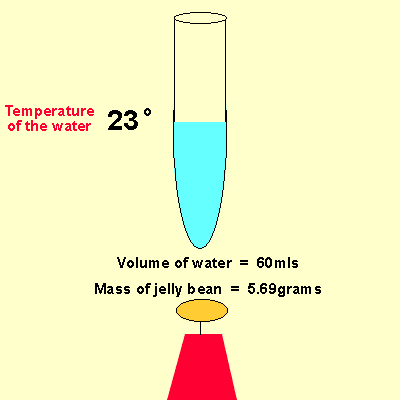Simple calorimetry Specific heat of water 4.18J/g/C° We can use the specific heat of water to make calculations on energy change during chemical reactions. Water has a specific heat capacity of 4.18J/g/C°. This means that 4.18 joules of energy must be supplied to every gram of water to raise the temperature by 1 degree Celsius.Look at the experiment above and calculate the amount of energy, in joules per gram of jelly bean. Step 1. Calculate the mass loss of the jelly bean. This is the amount that was burnt to release its energy. initital mass - final mass = 5.69 - 3.89 = 1.80 grams Step 2 Calculate the temperature rise initial -final = 28 - 23 =5°C Step 3 calculate the amount of energy released energy released = 4.18 X mass of water X temperature rise. energy released = 4.18 X 60 X 5 = 1254Joules or 1.25KJ Step 4 calculate the amount of energy per gram released by the jelly bean energy per gram = 1254 / 1.8 = 696.67Joules/gram Exercises